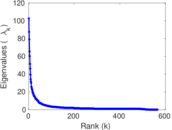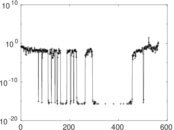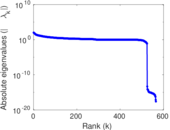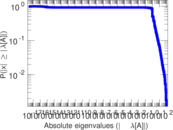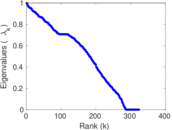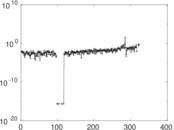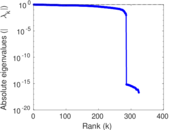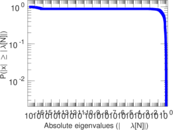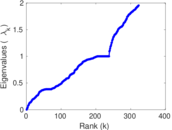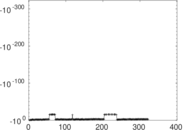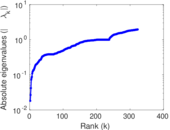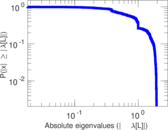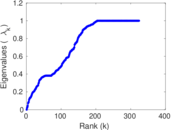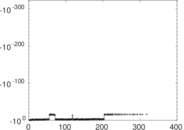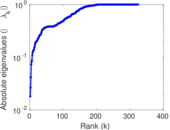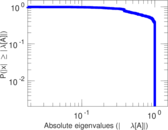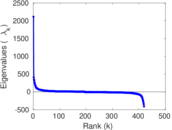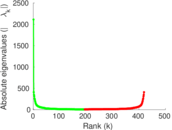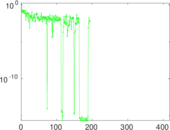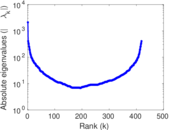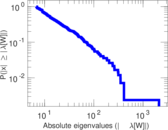# Wikipedia edits (ks)

This is the bipartite edit network of the Kashmiri Wikipedia. It contains users and pages from the Kashmiri Wikipedia, connected by edit events. Each edge represents an edit. The dataset includes the timestamp of each edit.

 Code `ks` Internal name `edit-kswiki` Name Wikipedia edits (ks) Data source http://dumps.wikimedia.org/ AvailabilityDataset is available for download Consistency checkDataset passed all tests Category Authorship network Dataset timestamp 2017-10-20 Node meaning User, article Edge meaning Edit Network formatBipartite, undirected Edge typeUnweighted, multiple edges Temporal dataEdges are annotated with timestamps

## Statistics

 Size n = 2,134 Left size n1 = 567 Right size n2 = 1,567 Volume m = 6,470 Unique edge count m̿ = 3,397 Wedge count s = 114,297 Claw count z = 4,167,577 Cross count x = 147,506,856 Square count q = 76,196 4-Tour count T4 = 1,074,574 Maximum degree dmax = 299 Maximum left degree d1max = 299 Maximum right degree d2max = 235 Average degree d = 6.063 73 Average left degree d1 = 11.410 9 Average right degree d2 = 4.128 91 Fill p = 0.003 823 35 Average edge multiplicity m̃ = 1.904 62 Size of LCC N = 1,540 Diameter δ = 15 50-Percentile effective diameter δ0.5 = 4.221 55 90-Percentile effective diameter δ0.9 = 6.895 60 Median distance δM = 5 Mean distance δm = 4.957 38 Gini coefficient G = 0.743 593 Balanced inequality ratio P = 0.197 450 Left balanced inequality ratio P1 = 0.156 414 Right balanced inequality ratio P2 = 0.236 321 Relative edge distribution entropy Her = 0.852 522 Power law exponent γ = 3.126 29 Tail power law exponent γt = 2.131 00 Tail power law exponent with p γ3 = 2.131 00 p-value p = 0.000 00 Left tail power law exponent with p γ3,1 = 1.841 00 Left p-value p1 = 0.696 000 Right tail power law exponent with p γ3,2 = 2.301 00 Right p-value p2 = 0.000 00 Degree assortativity ρ = −0.212 686 Degree assortativity p-value pρ = 4.788 93 × 10−36 Spectral norm α = 102.594 Algebraic connectivity a = 0.018 075 1 Spectral separation |λ1[A] / λ2[A]| = 1.172 95 Controllability C = 1,058 Relative controllability Cr = 0.502 374

## Plots

### Fruchterman–Reingold graph drawing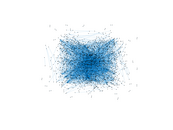### Degree distribution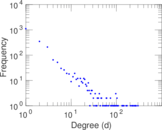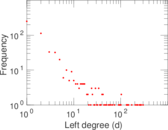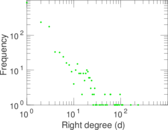### Cumulative degree distribution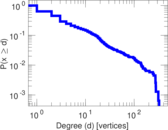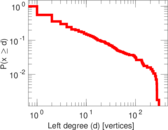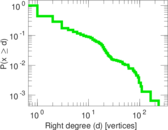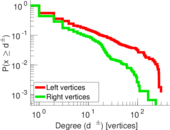### Lorenz curve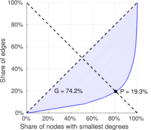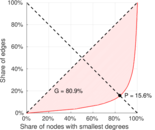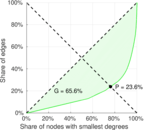### Spectral distribution of the adjacency matrix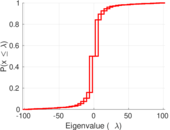### Spectral distribution of the normalized adjacency matrix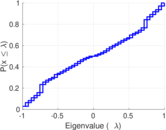### Spectral distribution of the Laplacian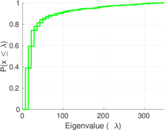### Spectral graph drawing based on the adjacency matrix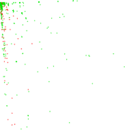### Spectral graph drawing based on the Laplacian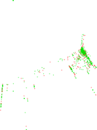### Spectral graph drawing based on the normalized adjacency matrix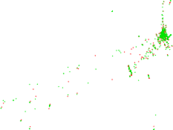### Degree assortativity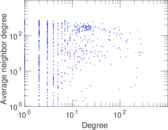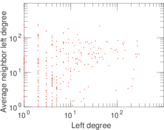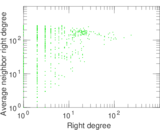### Zipf plot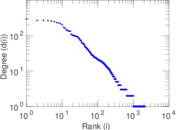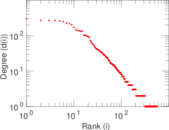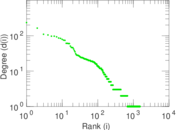### Hop distribution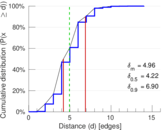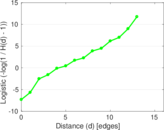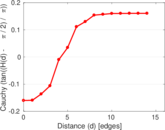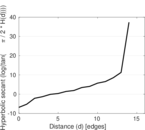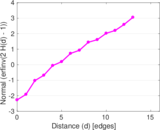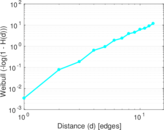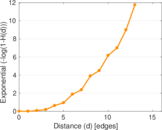### Double Laplacian graph drawing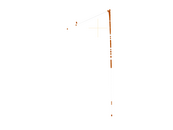### Delaunay graph drawing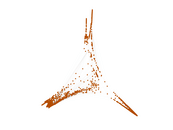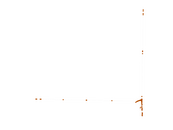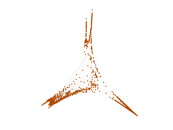### Edge weight/multiplicity distribution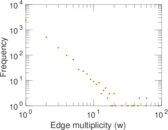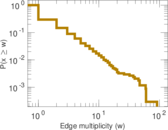### Temporal distribution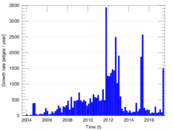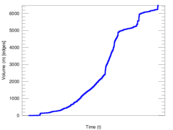### Temporal hop distribution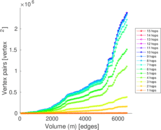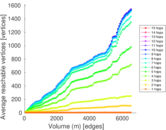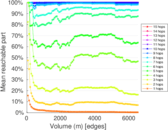### Diameter/density evolution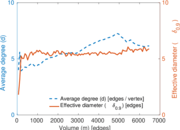### Matrix decompositions plots